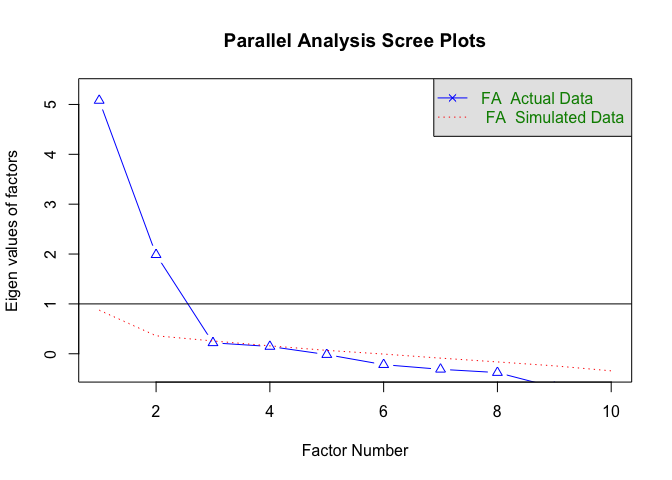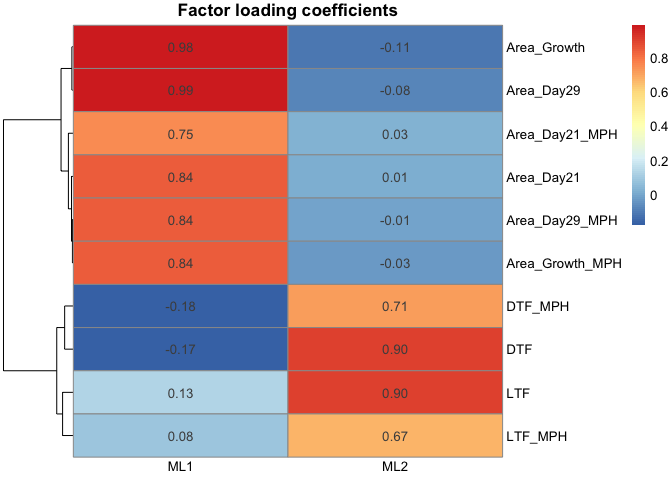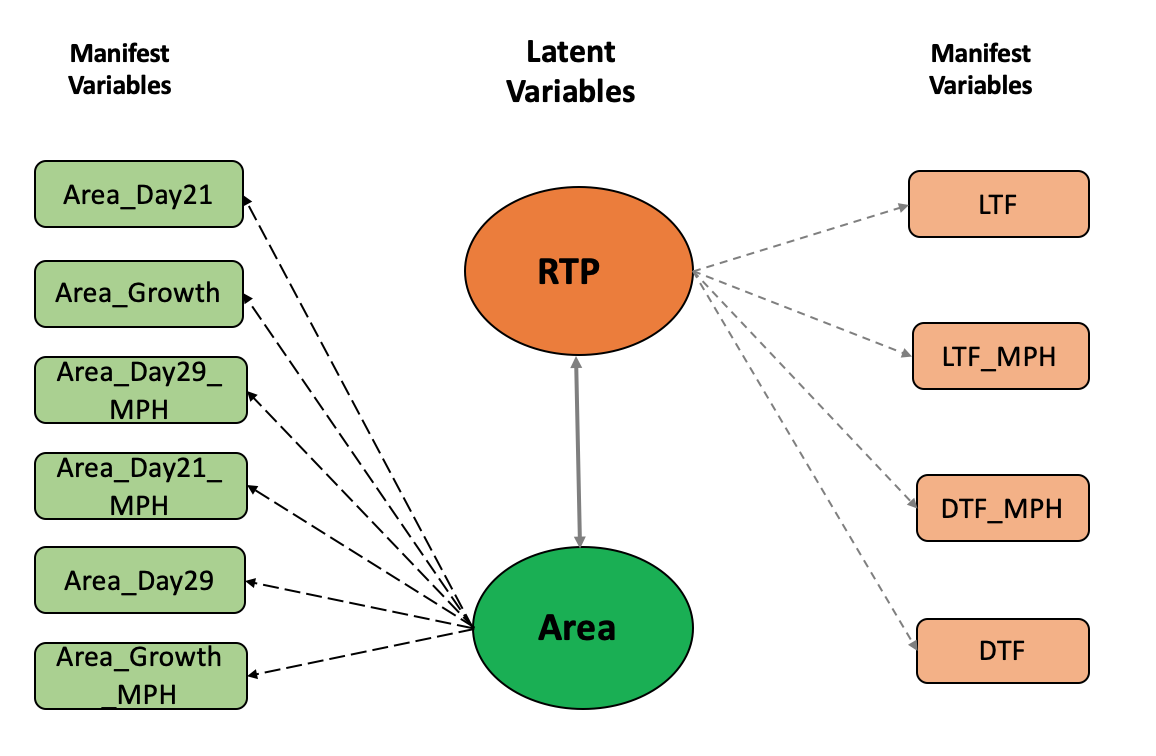# Factor Analytic Model for high-dimensional phenotypic data

## Background

Within complex traits system, traits are usually correlated due to the pleiotropic effects or linkage disequilibrium of QTLs. A multivariate mixed model is prevalent in animal and plant breeding considering it can impose the genetic or environmental covariance between traits. Nevertheless, there is a concern of computational efficiency when the number of traits is large, such as the high-dimensional phenotypes. Various approaches have been proposed to conquer computational challenges.

One of the proposed models is the factor analytic (FA) model, which represents the observed manifest variables using the underlying unobserved latent variables or factors by taking advantage of the mapping between them. In this tutorial, I introduced a factor analytic model, specifically, a combination of exploratory factor analysis (EFA) and confirmatory factor analysis (CFA) to decipher the high-dimensional phenotypes. Ten phenotypes (Area_Day21, Area_Growth, Area_Day29_MPH, Area_Day21_MPH, Area_Day29, Area_Growth_MPH, LTF, LTF_MPH, DTF, and DTF_MPH) from a public Arabidopsis data (Seymour et al. 2016) is selected and used to illustrate the FA model. The Arabidopsis data set used in this tutorial can be found in easyGWAS (Arabidopsis thaliana -> F1 Hybrids Seymour et. al. 2016, PNAS) or directly downloaded here.

library(blavaan)
library(dplyr)
library(knitr)
library(pheatmap)
library(psych)
library(tidyr)

## Data import

# import ten Arabidopsis phenotypes
dim(pheno) # 372  10
##  372  10

## Fit Exploratory Factor Analysis (EFA)

When there is no biological prior knowledge about the potential number of factors under the observed phenotypes, an exploratory factor analysis (EFA) could be exploited to search the underlying factors and the network structure between factors and phenotypes. Three steps have been implemented to fit the EFA: 1) identify the number of factors underlying observed phenotypes; 2) fit the EFA model using the number of factors detected in the first step; 3) initiate a clear network structure between factors and phenotypes using the factor loading coefficients derived from step 2.

### Step1: explore the number of factors under the phenotypes

# calculate phenotypic correlation between traits
COR <- cor(pheno, use = "complete.obs")

# parallel analysis to identify underlying factors using phenotypic correlation
fa.parallel(COR, n.obs = 123, fa = "fa", n.iter = 100, error.bars = FALSE,
se.bars = FALSE, ylabel = 'Eigen values of factors', fm = 'mle')## Parallel analysis suggests that the number of factors =  2  and the number of components =  NA

The general logic of parallel analysis is extracting the factors by comparing the eigenvalues of observed data and a data set simulated from observed data until observed data have a smaller eigenvalue than simulated data. The parallel scree plot suggested two factors underlying the observed phenotypes.

### Step2: fit EFA model using the number of factors (2) identified in step 1

# fit EFA model using maximum likelihood estimation
fit.mle <- fa(COR, nfactors = 2, fm = "ml", max.iter = 100,
rotate = "varimax")

pheatmap(Load, display_numbers = TRUE, cluster_cols = FALSE, angle_col = 0,
main = 'Factor loading coefficients', fontsize_number = 10)The factor loading heatmap implies the first factor (ML1) has a large contribution (factor loading coefficients) to Area associated traits, and the second factor (ML2) displays a large loading to the reproductive transition phase (RTP) traits (i.e., LTF, LTF_MPH, DTF_MPH, and DTF).

### Step 3: construct a clear network structure between factors and phenotypes by eliminating the cross-loadingsUsing the factor loading heatmap in step 2, a clear network structure between factors and observed phenotypes was constructed by eliminating the cross-loading (i.e., phenotype has factor loadings from more than one factor). This network structure was created by assigning observed phenotypes to corresponding factors with larger positive factor loading coefficients. Two biological names were assigned to the first and second factors as Area and reproductive transition phase based on the characteristic of the mapping between observed phenotypes and factors.

## Fit confirmatory factor analysis (CFA)

A confirmatory factor analysis (CFA) model requires a prior of underlying network structure between factors and observed phenotypes. Using the network structure constructed in EFA, a CFA model was fitted to estimate the factor scores of Area and RTP.

# create CFA model structure using the network structure identified in EFA model
CFA.Model <- '
# 6
Area  =~ Area_Day21 + Area_Growth + Area_Day29_MPH + Area_Day21_MPH +
Area_Day29 + Area_Growth_MPH
# 4
RTP =~ LTF + LTF_MPH + DTF_MPH + DTF
'

# fit the CFA modeling with bcfa function within blavaan package using the structure defined above
CFA.fit <- bcfa(CFA.Model, data = pheno,
burnin = 200, sample = 200, target = "stan",
save.lvs = T, n.chains = 2)

standardizedSolution(CFA.fit, type = 'std.lv', se = T) %>%
filter(op == "=~") %>%
select('Latent Variable' = lhs, 'Observed Phenotypes' = rhs,
'Estimate' = est.std, 'Posterior standard deviation' = se) %>%
kable(digits = 4, format = "html", caption = "Standardized Factor Loadings")
Latent Variable Observed Phenotypes Estimate Posterior standard deviation
Area Area_Day21 0.8460 0.0147
Area Area_Growth 0.9868 0.0013
Area Area_Day29_MPH 0.8392 0.0140
Area Area_Day21_MPH 0.7515 0.0214
Area Area_Day29 1.0000 0.0001
Area Area_Growth_MPH 0.8357 0.0152
RTP LTF 0.8765 0.0173
RTP LTF_MPH 0.6700 0.0347
RTP DTF_MPH 0.7381 0.0274
RTP DTF 0.9046 0.0179

### Estimate factor scores

bfs_all <- blavInspect(CFA.fit, 'lvmeans')
colnames(bfs_all) <- c('Area', 'RTP')
head(bfs_all)
##            Area          RTP
## [1,]  -1.315501  0.003476715
## [2,] -10.498799  0.028153987
## [3,]   4.791492 -0.009706926
## [4,]   3.090525 -0.020943780
## [5,]  -7.837373  0.023048771
## [6,]  -3.668090  0.009045961

The estimated factor scores of Area and Reproductive transiton phase could be considered as new phenotypes and used for further analysis. Be aware that I only used a small number of burn-in and Gibbs sampling for illustrative purposes. In practice, a larger number could be required according to the convergence check.